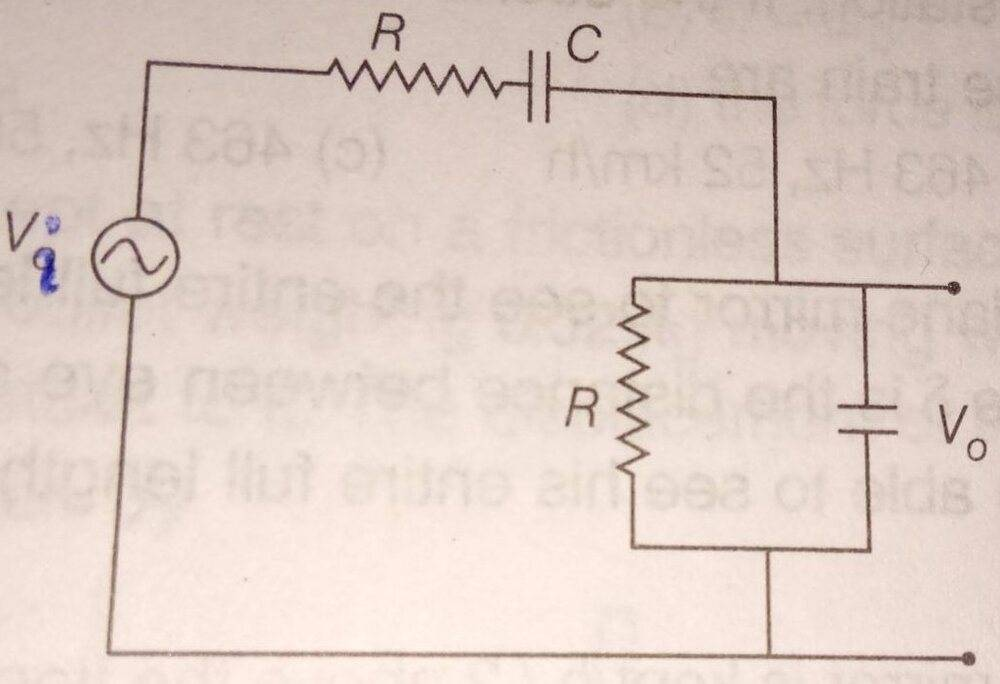# RC circuit using complex numbers

Homework Statement:
In the given RC network, for an input signal of angular frequency w=1/RC, the voltage gain |Vo|/|Vi| and the phase angle between Vo and Vi, respectively are:

1/2 and 0
1/3 and 0
1/2 and pi/2
1/3 and pi/2
Relevant Equations:
Xc = -j/(wC)
where j is the imaginary unit.

Kirchhof's Law:
Vi - IR - IXc - Vo = 0The impedance Z = R -j/wC + ##\frac{1}{\frac{1}{R} - \frac{\omega C}{j}}##
But,1/wC=R
So, solving this, I find:
Z= 3R/2(1-j)

|Z| =##\frac{3R}{\sqrt 2}##
I =##\frac{V_i \sqrt 2} {3R}##

Vi - IR-IXc =Vo

Solving this,
##Vo = V_i -\frac {V_i \sqrt 2}{3} - \frac{V_i \sqrt 2}{3R} \frac{-j}{wC}##
##Vo = V_i(1 -\frac {\sqrt 2}{3} - \frac{+j \sqrt 2}{3})##

This is something that doesn't match the options.

I think I am doing something conceptually wrong( like parallel addition of resistance and reactance maybe..). Please guide me.

Last edited by a moderator:

gneill
Mentor
You shouldn't be determining the current using the magnitude of the impedance. You'd want to find the complex current in order to retain phase information.

•Delta2, etotheipi and Kaguro
vela
Staff Emeritus
Homework Helper
Was the condition ##R = \frac{1}{\omega C}## given in the problem?

Was the condition ##R = \frac{1}{\omega C}## given in the problem?
Yes.

You shouldn't be determining the current using the magnitude of the impedance. You'd want to find the complex current in order to retain phase information.
Okay. I found the complex current!

So I wrote the Kirchhoff's law equation, this time using complex current. Then found that the imaginary terms cancelled and I end up with Vo=Vi/3.

So 0 phase difference between Vi and V0.
And Voltage gain is 1/3!

And that matched with the answer given.

Thank you very much!

With this, I have another question.

If the circuit is more complicated than this, with a series of multiple such parallel RC blocks plus series components,

Then if I am asked to find voltage drop across one of the parallel capacitor, I won't be able to write the Vo in terms of only series component voltage drop. How to find the Vo then?

•Delta2
DaveE
Gold Member
Usually more complex networks are most easily solved by using the Thevenin Source Transformations to reduce the complexity of the network. You may not have gotten that far in your studies yet though.

If you use KVL and KCL equations for big networks, you end up with lots of simultaneous equations to solve (i.e. big matrices). That works, especially if you are a computer simulator, but it's hard for us humans.

•Delta2 and Kaguro
Usually more complex networks are most easily solved by using the Thevenin Source Transformations to reduce the complexity of the network. You may not have gotten that far in your studies yet though.

If you use KVL and KCL equations for big networks, you end up with lots of simultaneous equations to solve (i.e. big matrices). That works, especially if you are a computer simulator, but it's hard for us humans.
Okay I'll look into it. Thank you once again.

hutchphd
Homework Helper
An "intermediate method" when there are isolated two port sections is to assign and calculate the complex impedance of each subunit. In this case one section has C parallel to R and one has C series R. The total impedance is these impedances in series.
Formally this is identical to @DaveE suggestion but sometimes this is quicker. It really means to chose the loops carefully!

•Kaguro and DaveE
DaveE
Gold Member
The broader point in circuit analysis is to get familiar with a set of tools that you can use to solve problems easily, by applying the best tools for the circumstances. There is a bit of art in STEM that involves recognizing which tools are best for the particular problem you have at hand. Often there are multiple paths to a solution, each with their own pros and cons.

•Kaguro and hutchphd
The broader point in circuit analysis is to get familiar with a set of tools that you can use to solve problems easily, by applying the best tools for the circumstances. There is a bit of art in STEM that involves recognizing which tools are best for the particular problem you have at hand. Often there are multiple paths to a solution, each with their own pros and cons.
Yes, such art exists in all the places, like the best technique to solve an integral.

All of this intuition comes from a lot of practice.

I've seen people using Laplace transform in electronics...

•Delta2
DaveE
•